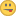# Future dates display as "less than one minute ago"

XMLWordPrintable

#### Details

•Bug
• Status: Closed
•Minor
• Resolution: Fixed
• 3.0.7-PL1, 3.1.0-dev
• None

#### Description

There are some cases when future dates display as less than a minute ago, which is really annoying for mods.
And also may affect phpbb on end of ban/poll dates.

Testing cases:
Set your date-format to something with || so it's shortened
than put something like:

 `echo \$user->format_date(time() - 30) . '
';` `echo \$user->format_date(time()) . '
';` `echo \$user->format_date(time() + 3600) . '
';` `echo \$user->format_date(time() + 7200) . '
';`

what you would expect is:

 `less than a minute ago` `less than a minute ago` `Today 11:21:16` `Today 12:21:16`

but what you get is:

 `less than a minute ago` `less than a minute ago` `less than a minute ago` `less than a minute ago`

the reason for this is, from the current code:

 ``` if (\$delta <= 3600 && (\$delta >= -5 || ((\$now / 60) % 60) == ((\$gmepoch / 60) % 60)) && \$date_cache[\$format]['is_short'] !== false && !\$forcedate && isset(\$this->lang['datetime']['AGO'])) ```

\$delta is -3600 and -7200 so both times <= 3600 and ((\$now / 60) % 60) == ((\$gmepoch / 60) % 60) is also true, as they are the same minute (doesn't care about the hour yet).

two possibilities I'd suggest:

• Remove detecting the minute, so it's only displayed as "less than a minute ago, when it is ago or the 5 seconds in future:

 `if (\$delta <= 3600 && (\$delta >= -5) && \$date_cache[\$format]['is_short'] !== false && !\$forcedate && isset(\$this->lang['datetime']['AGO']))`

• or if you want to keep the detecting of the whole minute (which I think is wrong, as it may still take 59 seconds in some cases) add a "\$delta > -60 &&" to the minute-check:

 `if (\$delta <= 3600 && (\$delta >= -5 || (\$delta > -60 && ((\$now / 60) % 60) == ((\$gmepoch / 60) % 60))) && \$date_cache[\$format]['is_short'] !== false && !\$forcedate && isset(\$this->lang['datetime']['AGO']))`

I'd prefer the first version.

#### PeopleToonArmy [X] (Inactive)Joas Schilling [X] (Inactive)